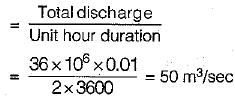Courses

# Hydrographs - 3

## 10 Questions MCQ Test Topicwise Question Bank for Civil Engineering | Hydrographs - 3

Description
This mock test of Hydrographs - 3 for Civil Engineering (CE) helps you for every Civil Engineering (CE) entrance exam. This contains 10 Multiple Choice Questions for Civil Engineering (CE) Hydrographs - 3 (mcq) to study with solutions a complete question bank. The solved questions answers in this Hydrographs - 3 quiz give you a good mix of easy questions and tough questions. Civil Engineering (CE) students definitely take this Hydrographs - 3 exercise for a better result in the exam. You can find other Hydrographs - 3 extra questions, long questions & short questions for Civil Engineering (CE) on EduRev as well by searching above.
QUESTION: 1

### A direct-runoff hydrograph due to a storm was found to be triangular in shape with a peak of 150 m3/s, time from start of effective storm to peak of 24 h and a total time base of 72 h. The duration of the storm in this case was

Solution:

The rising limb of a hydrograph, represents the increase in discharge due to the gradual building up of storage in channels and over the catchment surface. Basin and storm characteristics control the shape of the rising limb of a hydrograph. The recession limb is independent of storm characteristics and depends entirely on basin characteristics. Therefore, the duration of the storm in this case will be less than time from start of effective storm to peak i.e,, < 24 hr.

QUESTION: 2

Solution:
QUESTION: 3

### If a 4-h unit hydrograph of a catchment has a peak ordinate of 60 m3/s, the peak ordinate of an 8-h unit hydrograph for the same catchment will be

Solution:
QUESTION: 4

If the base period of a 6-hour hydrograph of a basin is 84 hours, then a 12 hours unit hydrograph derived from this 6 hour unit hydrograph will have a base period of

Solution:

The 12-hr Unit Hydrograph will be obtained by adding the ordinates of 6-hr and ordinates of 6-hr UH lagged by 6-hr and then dividing the ordinates by 2. So the base period for 12 hr UH will be, 84 + 6 = 90 hr.
Base period of derived UH,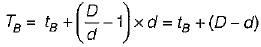where, tB is base period of known UH
D is duration of derived UH
d is duration of known UH.

QUESTION: 5

The peak discharges in 6 h and 12 h unit hydrographs of a catchment occur at t6 and f12 hours from the start. Then

Solution:
QUESTION: 6

Match List-I (Name of scientist) with List-II (Contribution to field of hydrology) and select the correct answer using the codes given below the lists: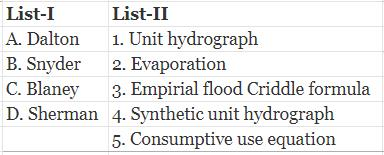Codes: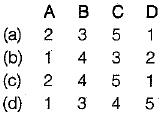Solution:
QUESTION: 7

Compactness coefficient of a basin is defined as

Solution:

Shape of a drainage basin is generally expressed by ‘form factor' and ‘compactness coefficient’.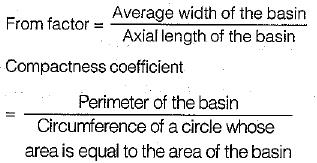QUESTION: 8

Unit hydrograph are generally used for
1. developing flood hydrographs for extreme rainfall magnitudes for the design of hydraulic structures.
2. extending flood flow records by using the available rainfall records.
3. developing flood forecasting and warning system based on rainfall data.

Solution:
QUESTION: 9

The direct runoff hydrograph of a storm obtained from a catchment is triangular in shape and has a base period of 80 hours. The peak flow rate is 30m3/sec and catchment area is 86.4 km2. The rainfall excess that has resulted the above hydrograph is

Solution: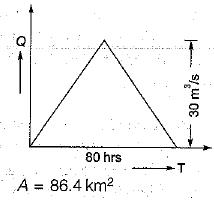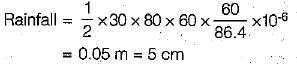QUESTION: 10

For a catchment area of 36 km2, the equilibrium discharge of an S-curve obtained by 2-hour unit hydrograph summation is

Solution:

Equilibrium discharge of S-curve